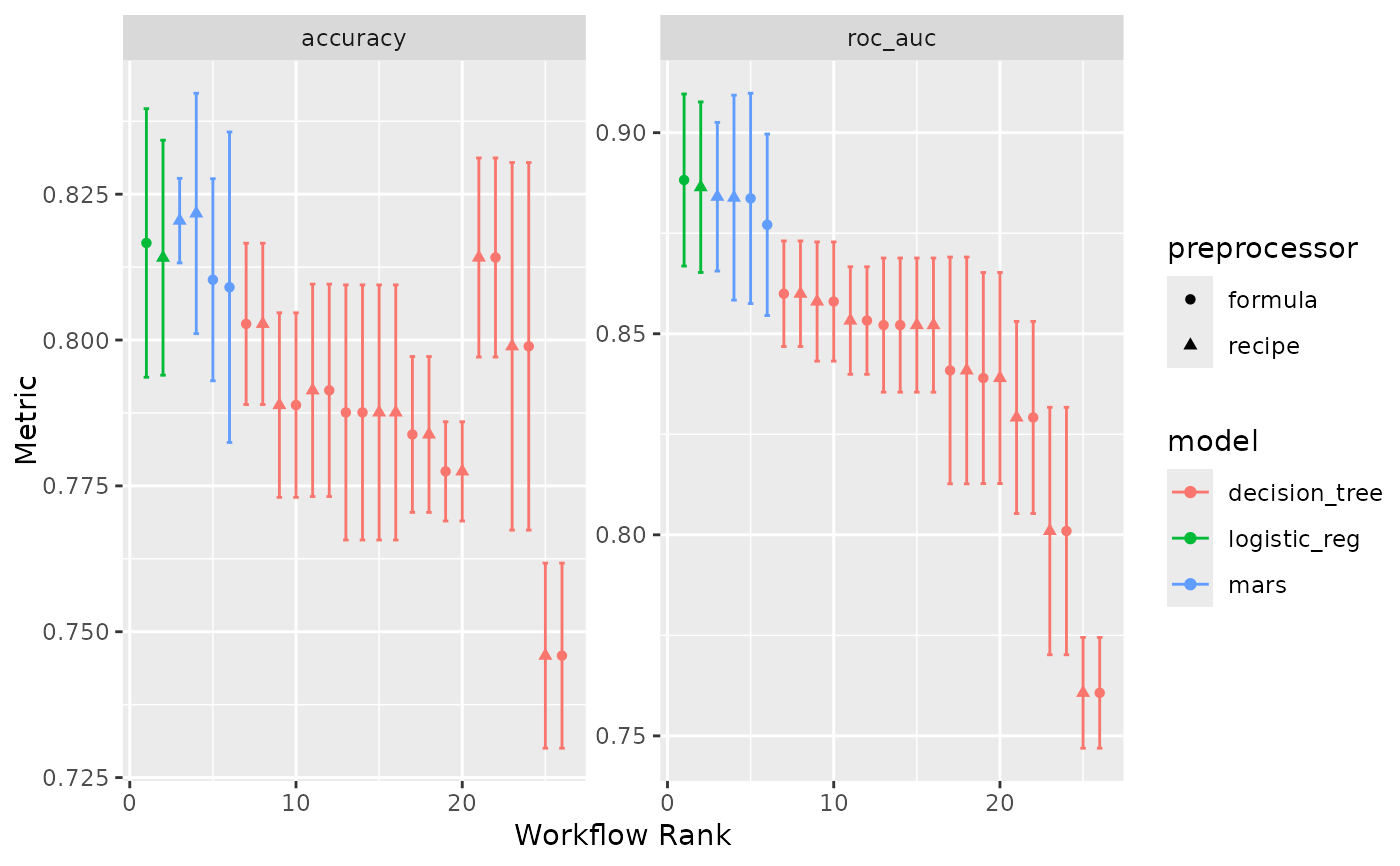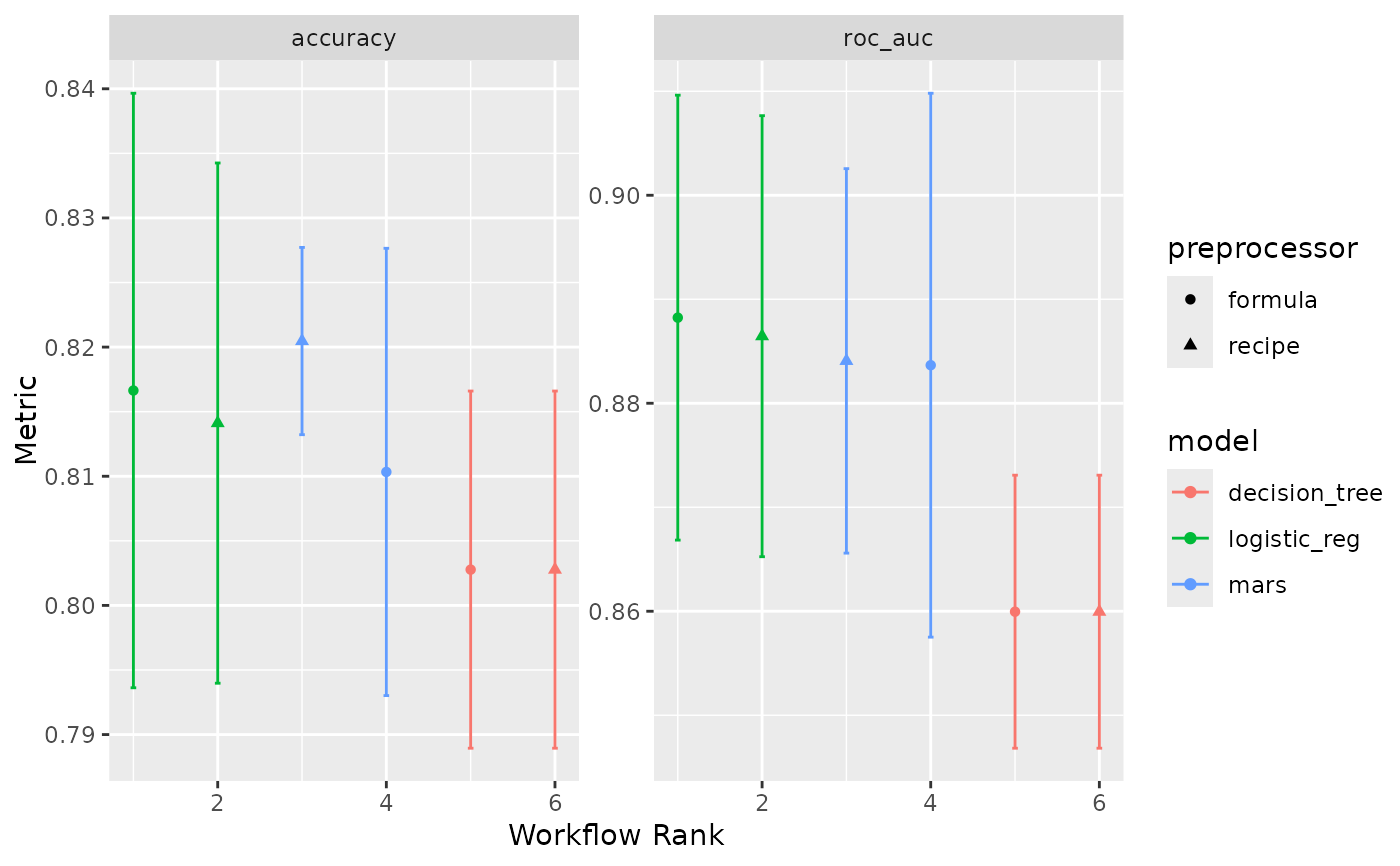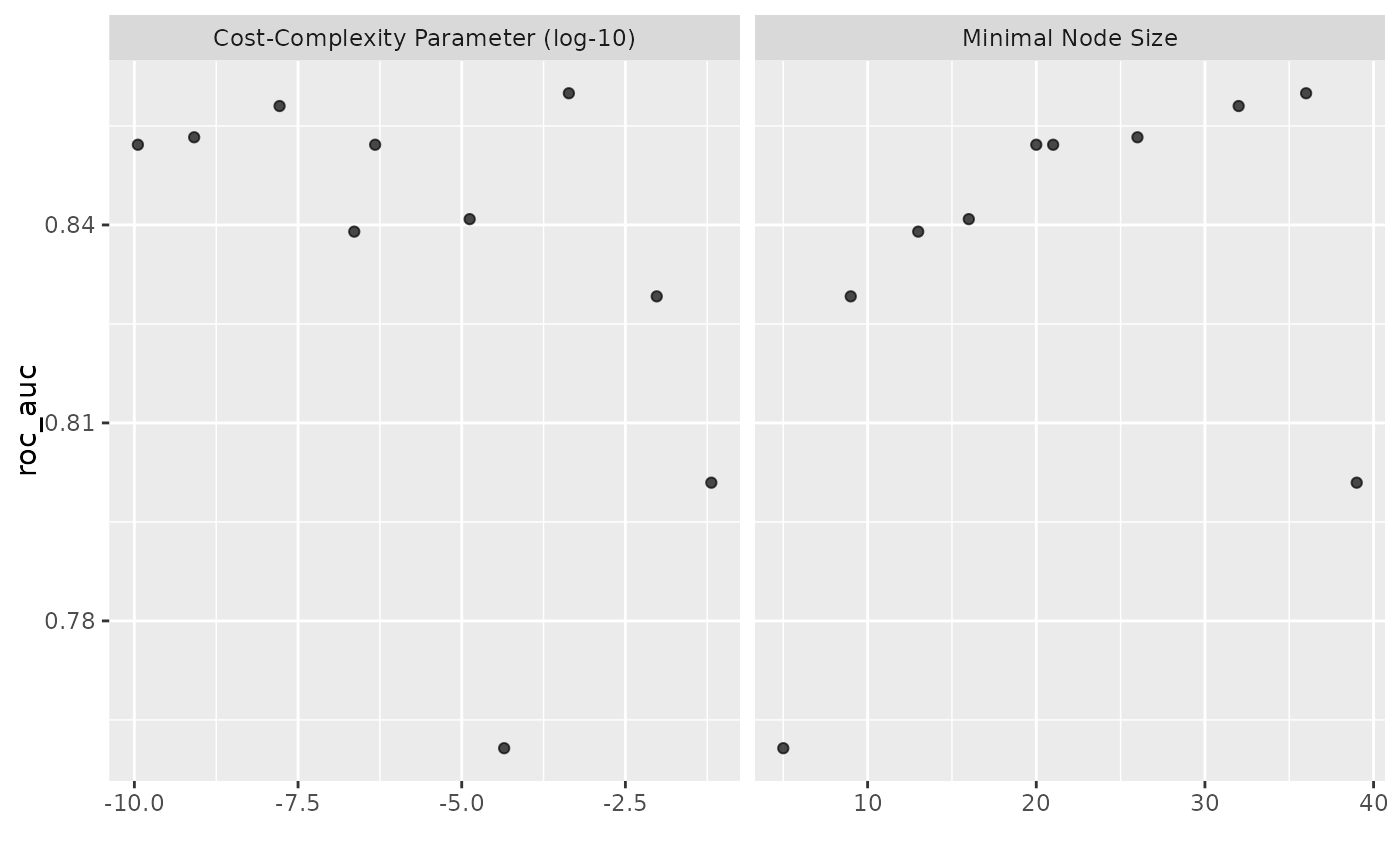This autoplot() method plots performance metrics that have been ranked using a metric. It can also run autoplot() on the individual results (per wflow_id).

## Usage

# S3 method for workflow_set
autoplot(
object,
rank_metric = NULL,
metric = NULL,
id = "workflow_set",
select_best = FALSE,
std_errs = qnorm(0.95),
...
)

## Arguments

object

A workflow_set whose elements have results.

rank_metric

A character string for which metric should be used to rank the results. If none is given, the first metric in the metric set is used (after filtering by the metric option).

metric

A character vector for which metrics (apart from rank_metric) to be included in the visualization.

id

A character string for what to plot. If a value of "workflow_set" is used, the results of each model (and sub-model) are ordered and plotted. Alternatively, a value of the workflow set's wflow_id can be given and the autoplot() method is executed on that workflow's results.

select_best

A logical; should the results only contain the numerically best submodel per workflow?

std_errs

The number of standard errors to plot (if the standard error exists).

...

Other options to pass to autoplot().

A ggplot object.

## Details

This function is intended to produce a default plot to visualize helpful information across all possible applications of a workflow set. A more appropriate plot for your specific analysis can be created by calling rank_results() and using standard ggplot2 code for plotting.

The x-axis is the workflow rank in the set (a value of one being the best) versus the performance metric(s) on the y-axis. With multiple metrics, there will be facets for each metric.

If multiple resamples are used, confidence bounds are shown for each result (90% confidence, by default).

## Note

The package supplies two pre-generated workflow sets, two_class_set and chi_features_set, and associated sets of model fits two_class_res and chi_features_res.

The two_class_* objects are based on a binary classification problem using the two_class_dat data from the modeldata package. The six models utilize either a bare formula or a basic recipe utilizing recipes::step_YeoJohnson() as a preprocessor, and a decision tree, logistic regression, or MARS model specification. See ?two_class_set for source code.

The chi_features_* objects are based on a regression problem using the Chicago data from the modeldata package. Each of the three models utilize a linear regression model specification, with three different recipes of varying complexity. The objects are meant to approximate the sequence of models built in Section 1.3 of Kuhn and Johnson (2019). See ?chi_features_set for source code.

## Examples

autoplot(two_class_res)autoplot(two_class_res, select_best = TRUE)autoplot(two_class_res, id = "yj_trans_cart", metric = "roc_auc")